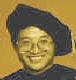Differential Equations and Computational Simulations III
Electron. J. Diff. Eqns., Conf. 01, 1997, pp. 193--199.

### An inverse problem in a parabolic equation Zhilin Li & Kewang Zheng

Abstract:
In this paper, an inverse problem in a parabolic equation is studied. An unknown function in the equation is related to two integral equations in terms of heat kernel. One of the integral equations is well-posed while another is ill-posed. A regularization approach for constructing an approximate solution to the ill-posed integral equation is proposed. Theoretical analysis and numerical experiment are provided to support the method.

Published November 12, 1998.
Mathematics Subject Classifications: 35K05, 65R30.
Key words and phrases: Coefficients identification, ill-posedness, regularization.

Show me the PDF file (106K), TEX file, and other files for this article.Zhilin Li Department of Mathematics and Center for Research in Scientific Computation North Carolina State University Raleigh, NC 27695-8205 USA E-mail address: zhilin@math.ncsu.edu Kewang Zheng Department of Mathematics Hebei University of Science & Technology Hebei 050018, China